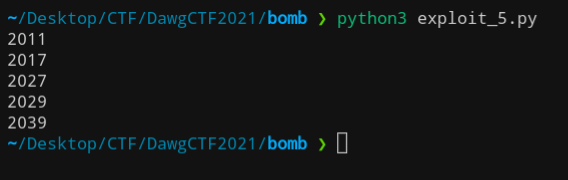# Phase 5 (150 pts)

Getting more spicy...

## Problem Statement​

``Are you really, really ready and excited for this stage?(Flag uses the same rockyou.txt format as BBomb Phase 4)Update: No input numberis below 2010 and each number increasingly larger than the previousAuthor: treap_treap``

## Analysing​

Let's take a look at what Ghidra tells us. There is a function which I have named prime because that is exactly what it does.

``uint phase5(undefined8 param_1,undefined4 param_2,undefined4 param_3,undefined4 param_4,           undefined4 param_5,undefined4 param_6,undefined4 param_7,undefined4 param_8,char *param_9           ){  void *inputPtr;  undefined8 uVar1;  undefined4 extraout_XMM0_Da;  uint result;  int sum;  int counter;    puts("\nAre you really, really ready and excited for this stage?");  result = 1;  inputPtr = calloc(4,4);  getInput(extraout_XMM0_Da,param_2,param_3,param_4,param_5,param_6,param_7,param_8,5,param_9,           "%d%d%d%d",inputPtr,(long)inputPtr + 4,(long)inputPtr + 8,(char)inputPtr + '\f');  sum = 0;  counter = 0;  while (counter < 4) {    if ((0 < counter) && ((int)param_9[(long)counter + -1] < param_9[counter] + -10)) {      result = 0;    }    uVar1 = prime(*(uint *)((long)inputPtr + (long)counter * 4));    result = result & (uint)uVar1;    sum = sum + *(int *)((long)inputPtr + (long)counter * 4);    counter = counter + 1;  }  if (sum != 0x1f94) {    result = 0;  }  free(inputPtr);  return result;}``

### Solve.py​

We can see from the decompiler that every integer must be greater than the previous and the program accepts 4 different numbers.

``for i in range(2010, 2050):    prime = True    if (i & 1 == 0):        continue    for j in range(3, i // 2 + 1, 2):        if (i % j == 0):            prime = False            break    if (prime):        print(i)``

### Output​We have to choose 4 which adds up to `0x1f94` which equates to the decimal value of `8084`.

Hence, the values are: 2011, 2017, 2027, 2029.

## Flag​

`DawgCTF{kayla1_harris_ilovemike_valencia}`